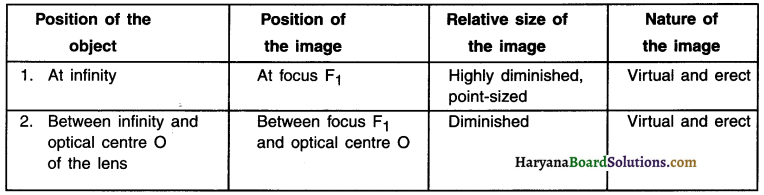# HBSE 10th Class Science Solutions Chapter 10 Light Reflection and Refraction

Haryana State Board HBSE 10th Class Science Solutions Chapter 10 Light Reflection and Refraction Textbook Exercise Questions and Answers.

## Haryana Board 10th Class Science Solutions Chapter 10 Light Reflection and Refraction

HBSE 10th Class Science Light Reflection and Refraction Textbook Questions and Answers

Question 1.
Which one of the following materials cannot be used to make a lens?
(a) Water
(b) Glass
(c) Plastic
(d) Clay
(d) ClayQuestion 2.
The Image formed by a concave mirror is observed to be virtual, erect and larger than the object. Where should be the position of the object?
(a) Between the principal focus and the centre of curvature
(b) At the centre of curvature
(c) Beyond the centre of curvature
(d) Between the pole of the mirror and its principal focus.
(d) Between the pole of the mirror and its principal focus.

Question 3.
Where should an object be placed in front of a convex lens to get a real image of the size of the object?
(a) At the principal focus of the lens
(b) At twice the focal length
(c) At Infinity
(d) Between the optical centre of the lens and its principal focus.
(b) At twice the focal length

Question 4.
A spherical mirror and a thin spherical lens have each a focal length of -15 cm. The mirror and the lens are likely to be …….
(a) both concave
(b) both convex
(c) the mirror Is concave and the lens is convex
(d) the mirror is convex, but the lens is concave
(a) both concaveQuestion 5.
No matter how far you stand from a mirror, your image appears erect. The mirror is likely to be …………
(a) plane
(b) concave
(c) convex
(d) either plane or convex
(d) either plane or convex

Question 6.
Which of the following lenses would you prefer to use while reading small letters found in a dictionary?
(a) A convex lens of focal length 50 cm
(b) A concave lens of focal length 50 cm
(c) A convex lens of focal length 5 cm
(d) A concave lens of focal length 5 cm
(c) A convex lens of focal length 5 cm

Question 7.
We wish to obtain an erect image of an object, using a concave mirror of focal length 15 cm. What should be the range of distance of the object from the mirror? What is the nature of the image? Is the image larger or smaller than the object? Draw a ray diagram to show the image formation in this case.
1. When the object is placed between the pole and the principal focus of the concave mirror, we get an erect, virtual and enlarged image.
2. We are given that the focal length of concave mirror = 15 cm
3. So, the object should be placed in front of the concave mirror at a distance less than 15 cm.

Question 8.
Name the type of mirror used in the following situations.
(b) Side/rear-view mirror of a vehicle
(c) Solar furnace
(a) Concave mirrors are used as reflectors in headlights of cars. When a bulb is located at the focus of the concave mirror, the light rays after reflection from the mirror travel over a large distance as a parallel beam of high intensity.

(b) A convex mirror is used as a side/rear-view mirror of a vehicle because of the following reasons:

• A convex mirror always forms an erect, virtual and diminished image of an object placed anywhere in front of it.
• A convex mirror has a wider field of view than a plane mirror of the same size.

(c) Large concave mirrors are used to concentrate sunlight to produce heat in solar furnaces. Concave mirrors are able to raise the temperature drastically.Question 9.
One-half of a convex lens is covered with a black paper. Will this lens produce a complete image of the object? Verify your answer experimentally. Explain your observations.
As shown in figure, when the lower half of the convex lens is covered with a black paper, it still forms the complete image of the object, but the intensity of the image is reduced.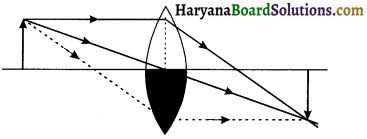The rays coming from the object get refracted by the upper half of the lens and meet at the other side of the lens to form the image.

Question 10.
An object 5 cm in length is held 25 cm away from a converging lens of focal length 10 cm. Draw the ray diagram and find the position, size and the nature of the Image formed.
The final position, nature and size of the image A’B’ are:
Here, Object size h = +5 cm
Object distance u = – 25 cm
Focal length of lens f = + 10 cm
( ∵ Converging, i.e., Convex lens)
Image distance y =?
Image height h’ =?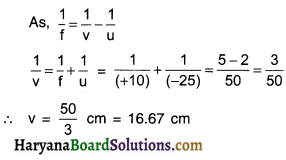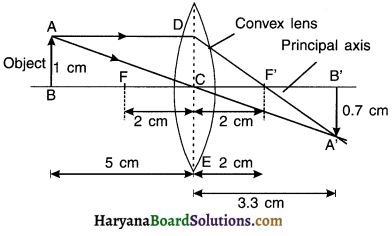Image distance y is positive. This shows that the image formed is real and on the other side of the lens, at 16.67 cm from the lens as shown in the figure. AB : Object, A’B’: Image
Now, Magnification m = $$\frac{h^{\prime}}{h}=\frac{v}{u}$$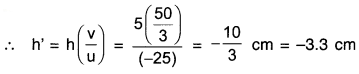The negative (minus) sign shows that the image is inverted, real, diminished (3.3 cm). Figure 10.60 show the positive, size and nature of the image formed.Question 11.
A concave lens of focal length 15 cm forms an Image 10 cm from the lens. How far is the object placed from the lens? Draw the ray diagram.
Scale: 1 cm = 5 cm
Focal length, f = – 15 cm [f is – ve for a concave lens]
Image distance, v = – 10 cm
[Concave lens forms virtual image on same side as the object, so v is – ve]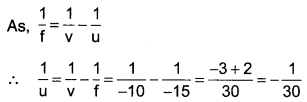Object distance, u = – 30 cm.
The negative sign shows that the object is placed on the left side of the lens.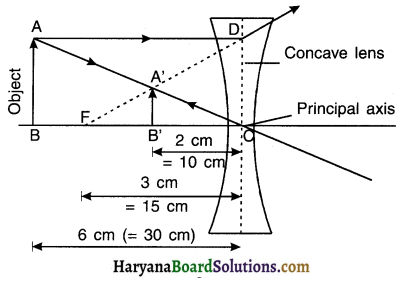Question 12.
An object Is placed at a distance of 10 cm from a convex mirror of focal length 15 cm. Find the position and nature of the image.
Object distance, u = – 10 cm
Focal length, f = + 15 cm [f is +ve for a convex mirror]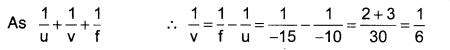Image distance, v = + 6 cm.
As v is +ve, so a virtual, erect image is formed at a distance 6 cm behind the mirror.

Question 13.
The magnification produced by a plane mirror is +1. What does this mean?
Magnification of a mirror $$m=\frac{h^{\prime}}{h}=\frac{-v}{u}$$
Here, for a plane mirror, m = + 1, So, h’ = h and y = – u

• m = 1 indicates that both ¡mage and object are of same size.
• Positive sign of m indicates that a virtual image is formed behind the mirror.Question 14.
An object 5.0 cm in length is placed at a distance of 20 cm in front of a convex mirror of radius of curvature 30 cm. Find the position of the image, its nature and size.
Object size, h = + 5cm
Object distance, u = – 20 cm
Radius of curvature, R = + 3.0 cm [R is +ve for a convex mirror]
Focal length, $$f=\frac{R}{2}$$ = + 15 cm
From mirror formula,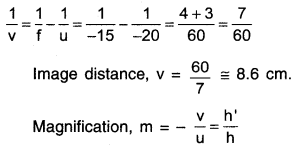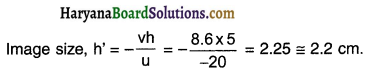A virtual, erect image of height 2.2 cm is formed behind the mirror at a distance of 8.6 cm from the mirror.

Question 15.
An object of size 7.0 cm Is placed at 27 cm in front of a concave mirror of focal length 18 cm. At what distance from the mirror should a screen be placed, so that a sharply focused image can be obtained? Find the size and the nature of the Image.
Object size, h = + 7.0 cm
Focal length, t = – 18 cm
Image size, h’ = ?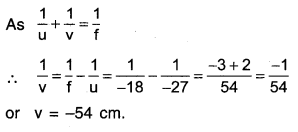Object distance, u = – 27 cm
image distance,v = ?
The screen should be placed at a distance of 54 cm on the object side of the mirror to obtain sharp image.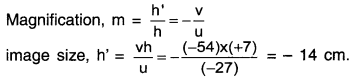The image is real, inverted and enlarged in size.Question 16.
Find the focal length of a lens of power – 2.0 D. What type of lens is this?
Here, P = – 2.0 D f =$$\frac{1}{P}=\frac{1}{-2.0 D}$$ = – 0.5 m.
Since the power of lens is negative, the lens is concave.

Question 17.
A doctor has prescribed a corrective lens of power +1.5 D. Find the focal length of the lens. Is the prescribed lens diverging or converging?
Here P = +1.5D
$$f=\frac{1}{P}=\frac{1}{+1.5 D}=+\frac{10}{15} m$$ = + 66.67cm.

• As the focal length is positive, the prescribed lens is converging.
• Opposite side at F, real and inverted, highly diminished (point sized)

HBSE 10th Class Science Light Reflection and Refraction InText Activity Questions and Answers

Textbook Page no – 168

Question 1.
Define the principal focus of a concave mirror.
The point (F) on the principle axis where the beam of light parallel to the principle axis either actually converges to or diverges from, after reflection from a mirror is called the principle focus (F).

Question 2.
The radius of curvature of a spherical mirror is 20 cm. What is its focal length?
R = 20cm.
Focal length (f) = $$\frac{\mathrm{R}}{2}=\frac{20}{2}$$ = 10 cm

Question 3.
Name a mirror that can give an erect and enlarged image of an object.
Concave mirrorQuestion 4.
Why do we prefer a convex mirror as a rear-view mirror in vehicles?
A convex mirror is preferred as a rear-view mirror because —

• Convex mirror always forms a virtual, erect and a diminished (point-like) image of an object placed anywhere in front of it. This means the driver can see the image of person even at a very distant position.
• Convex mirror has a wider field of view. So it covers a large rear distance. This helps the driver as he can see more.

Textbook Page no – 171

Question 1.
Find the focal length of a convex mirror whose radius of curvature is 32 cm.
The radius of curvature and focal length of a convex mirror are positive.
∴ R = + 32cm and f = $$\frac{\mathrm{R}}{2}$$ = + 16cm

Question 2.
A concave mirror produces three times magnified (enlarged) real Image of an object placed at 10 cm in front of It. Where is the image located?
As the image is real, so magnification m must be negative.
$$m=\frac{h^{\prime}}{h}=-\frac{v}{u}=-3$$
∴ v = 3 x (-10) = – 30 cm.
Thus the image is real and it is located at a distance of 30 cm from the mirror in the front of mirror.

Textbook Page no – 176

Question 1.
A ray of light travelling in air enters obliquely into water. Does the light ray bend towards the normal or away from the normal? Why?
1. Light travels faster in rarer medium air and slower in denser medium water.
2. Since the ray of light travelling in air enters obliquely into water, it slows down and bends towards the normal.

Question 2.
Light enters from air to glass having refractive Index 1.50. What is the speed of light in the glass? The speed of light in vacuum is 3 x 108 mr1.
1. Refractive index of glass, ng = 1.50
Speed of light in vacuum, c = 3 x 108 ms-1

2. Absolute refractive index of glass, ng = ((c/v))
∴ Speed of light in glass $$v=\frac{c}{n_g}=\frac{3 \times 10^8 \mathrm{~m} / \mathrm{s}}{1.5}=2 \times 10^8 \mathrm{~ms}^{-1}$$Question 3.
Find out, from table 10.3 of the text book, the medium having highest optical density. Also find the medium with lowest optical density.
1. From table 10.3, diamond has highest refractive index (= 2.42), so it has largest optical density.
2. Air has lowest refractive index (= 1.0003), so it has lowest optical density.

Question 4.
You are given kerosene, turpentine and water. In which of these does the light travel fastest? Use the information given In table 10.3 of the text book.
For kerosene, n = 1. 44,   For turpentine oil, n = 1. 47,   For water, n = 1. 33
As water has lowest refractive index, so light travels fastest in this optically rarer medium compared to kerosene and turpentine oil.

Question 5.
The refractive index of diamond is 2.42. What is the meaning of this statement?
It indicates that the ratio of speed of light in air to that in diamond is 2.42.

Textbook Page no – 184.

Question 1.
Define 1 dioptre of power of a lens.
1 dioptre (1 D) is the power of a lens whose focal length is 1 meter.

Question 2.
A convex lens forms a real and inverted image of a needle at a distance of 50 cm from it. Where is the needle placed ¡n front of the convex lens if the image is equal to the size of the object? Also, find the power of the lens.
1. Here, image distance y = + 50 cm (∴ y is positive for real images)
2. As mentioned, the real image is of the same size as the object.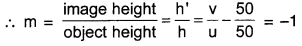Thus, object (needle) is placed at 50 cm from the lens.

Power of lens: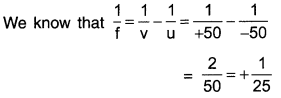∴ Focal length (f) = 25 cm = 0.25 m
Now, $$P=\frac{1}{f}=\frac{1}{+25}=+4 D$$
Thus, power of lens = + 4D

Question 3.
Find the power of a concave lens of focal length 2 m.
Since the focal length of a concave lens is negative; f = – 2m.
Power $$P=\frac{1}{f}=\frac{1}{-2 m}=-0.5 \mathrm{D}$$Activities

Activity 1.

Aim: To study the formation of images by both sides of a large shining spoon.

Apparatus required: A large shining spoon.

Procedure:

• Take a large shining spoon. Try to view face in its curved surface.
• Do you get the image? Is it smaller or larger?
• Move the spoon slowly away from your face. Observe the Image. How does it change?
• Reverse the spoon and repeat the activity. How does the image look like now?
• Compare the characteristics of the image on the two surfaces.

Observation and Conclusion:

• Initially, we can see our tace inside the curved portion of the spoon. The image of our face looks erect and enlarged. As we move the spoon at a farther distance, the image goes on becoming smaller and then gets inverted. The inner portion of the spoon behaves as a concave mirror.
• When we see the spoon from outer surface, it behaves as a convex mirror. The image formed is erect and small Irrespective of the distance of face from the spoon.

Activity 2.

Aim: To find the approximate focal length of a concave mirror.

Apparatus required: Concave mirror, a sheet of paper and meter scale

Caution: Do not look at the sun directly or even Into a mirror reflecting sunlight. It may damage your eyes.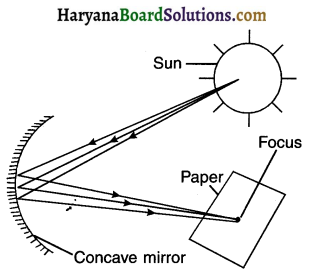Procedure:

• Hold a concave mirror in your hand and direct its reflecting surface towards the sun.
• Direct the light reflected by the mirror onto a sheet of paper held close to the mirror.
• Move the sheet of paper back and forth gradually unto you find on the paper sheet a bright, sharp spot of light

Question 1.
Hold the mirror and the paper in the same position for a few minutes. What do you observe? Why?
Observation
Initially, the paper turns blackish and emits smoke while burning slowly. The paper then catches fire.

Conclusion:

• The point at which the sun-rays get converged to produce tire is the principal focus (F) of the concave mirror.
• The distance of this image of sun at point F gives the approximate value of focal length (t) of the concave mirror.Activity 3.

Aim: To locate the image formed by a concave mirror for different positions of the object.

Procedure:
1. Take a concave mirror. Find out its approximate focal length. Let f = 10 cm.

2. Mark a line on a table with a chalk. Place the concave mirror on a stand. Place the stand over the line such that its pole lies over the line.

3. Draw with a chalk two more lines parallel to the previous line such that the distance between any two successive lines is equal to the focal length of the mirror. These lines will correspond to the position of the points P, F and C, respectively.

4. Keep a bright object, say a burning candle, at a position far beyond C. Place a paper screen and move it in front of the
mirror till you obtain a sharp bright image of the candle flame on it.

5. Observe the image carefully. Note down its nature, position arid relative size with respect to the object size.

6. Repeat the experiment by placing the candle —
(a) just beyond C, (b) at C, (C) between F and C, (d) at F, and (e) between P and F

7. In one of the cases, you may not get the image on the screen. Identify the position of the object in such a case. Then, look for its virtual image in the mirror itself.

8. Note down and tabulate your observations.

Observation:
Table 1. Nature, size and position of Images formed by a concave mirror for different positions of the object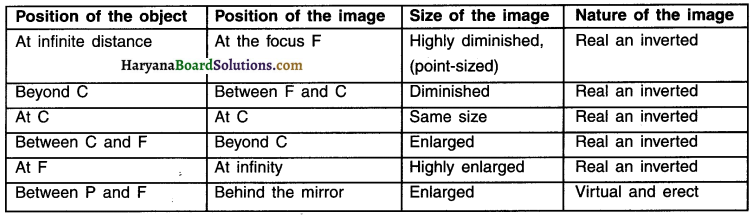Activity 4.

Students should do this activity on their own. Make use of activity 3 for drawing the diagrams.

Activity 5.

Aim: To locate the image formed by a convex mirror for different positions of the object.

Take a convex mirror. Hold it in one hand. Hold a pencil in the upright position in the other hand.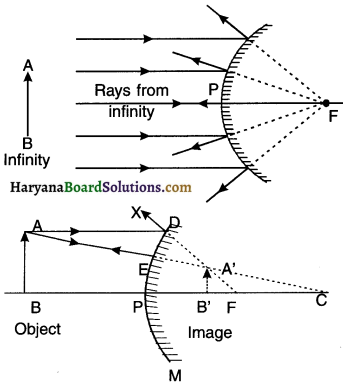Question 1.
Observe the image of the pencil in the mirror. Is the image erect or inverted? Is it diminished or enlarged?
The image is erect, diminished and virtual.

Question 2.
Move the pencil away from the mirror slowly. Does the image become smaller or larger?
The image formed is smaller and erect.

Question 3.
Repeat this activity carefully. State whether the image will move closer to or farther away from the focus as the object is moved away from the mirror?
As the object is moved away from the mirror, the image moves closer to the focus of the mirror.Observation: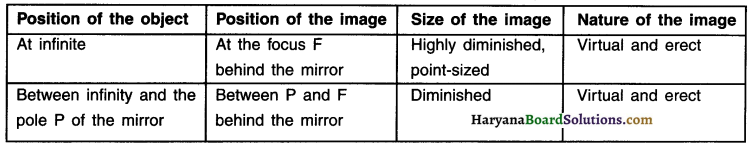Activity 6.

Aim: To demonstrate that convex mirror has a wide field of view.

Question 1.
Observe the Image of a distant object, say a distant tree, in a plane mirror. Could you see a full-length Image?
No, we cannot see the full-length image of the object in plane mirror.

Question 2.
Try with plane mirrors of different sizes. Did you see the entire object In the Image?
When we try with plane mirrors of different sizes, we find that the entire object in the image is seen only when the size of the plane mirror is at least half the size of the object.

Question 3.
Repeat this activity with a concave mirror. Did the mirror show full length Image of the object?
The full length image is not formed even in concave mirror.

Question 4.
Now try using a convex mirror. Did you succeed? Explain your observations with reason.
A full length image of the object is obtained in a small convex mirror.

Reasons for these observations:

• In a plane mirror, size of image is always equal to the size of the object.
• In case of a concave mirror, when the object is between P and F only then we get an enlarged, virtual
and erect image behind the mirror. But here, since the object is far away, its full length image cannot be seen.
• In a convex mirror, the image is always virtual, erect and smaller than the object.

Activity 7.

Aim: To demonstrate refraction of light.

1. Place a coin at the bottom of a bucket filled with water.
2. With your eye to a side above water, try to pick up the coin in one go. Did you succeed in picking up the coin?
3. Repeat the activity. Why did you not succeed in doing in one go?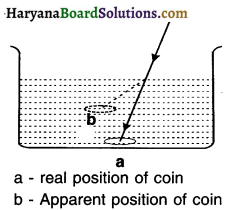Observation and conclusion:

• Due to refraction of light from water, the coin appears to be slightly above its actual position.
• This visual illusion makes it difficult to identify exact position of the coin and pick it. However, after trying a while we can pick it up.

Activity 8.

1. Place a large shallow bowl on a table and put a coin in it.
2. Move away slowly from the bowl. Stop when the coin just disappears from your sight.
3. Ask a friend to pour water gently into the bowl without disturbing the coin.
4. Keep looking for the coin from your position. Does the coin becomes visible again from your position? How could this happen?

Observation and Conclusion: On pouring water into the bowl, the coin becomes visible again. Moreover, due to refraction of light, the coin appears slightly raised above its actual position. This happens due to refraction of light.Activity 9.

1. Draw a thick straight line in ink, over a sheet of white paper placed on a table.
2. Place a glass slab over the line in such a way that one of its edges makes an angle with the line.

Question 1.
Look at the portion of the line under the slab from the sides. What do you observe? Does the line under the glass slab appear to be bent at the edges?
We see the line first in the air medium and then in the glass. So, the line under the glass slab appears bent at the edges-due to refraction.

Question 2.
Next, place the glass slab such that it ¡s normal to the line. What do you observe now? Does the part of the line under the glass slab appear bent?
Now, the line does not appear bent. The reason for this is that the light rays incident normally at the glass and air do not undergo refraction.

Question 3.
Look at the line from the top of the glass slab. Does the part of the line, beneath the slab, appear to be raised? Why does this happen?
Yes, part of the line under the glass slab appears to be raised. This is due to the refraction of light rays when they travel from glass to air.

Activity 10.

1. Fix a sheet of white paper on a drawing board using drawing pins.
2. Place a rectangular glåss slab over the sheet in the middle.
3. Draw the outline of the slab with a pencil. Let us name the outline as ABCO.
4. Take four identical pins.
5. Fix two pins, say E and F, vertically such that the line joining the pins is inclined to the edge AB.
6. Look for the images of the pins E and F through the opposite edge. Fix two other pins, say G and H, such that these pins and the images of E and F lie on a straight line.
7. Remove the pins and the slab.
8. Join the positions of tip of the pins E and F and produce the line up to AB. Let EF meet AB at O. Similarly, join the positions of tip of the pins G and H and produce it up to the edge CD. Let HG meet CD at O’.
9. Join O and O’. Also produce EF up to P, as shown by a dotted line in Figure.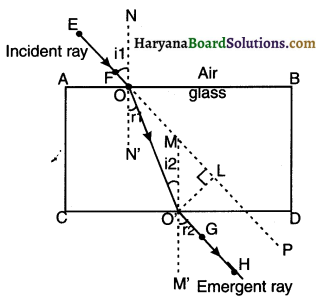Activity 11.

Aim: To show the converging nature of convex lens.
Caution: Do not look at the Sun directly or through a lens while doing this Activity or otherwise. You may damage your eyes if you do so.

Procedure:

• Hold a convex lens in your hand. Direct it towards the Sun.
• Focus the light from the Sun on a sheet of paper. Obtain a sharp bright image of the Sun.
• Hold the paper and the lens in the same position for a while. Keep observing the paper. What happens? Why? Recall your experience in Activity 2.Observation and conclusion:

• The paper begins to burn producing smoke. Later, it catches fire.
• We conclude that the light rays from the sun gets converged when they pass through the lens and form a bright spot on the paper. This spot shows the focus of the lens.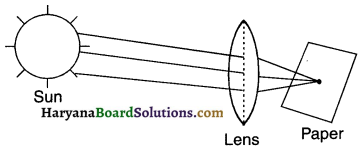Activity 12.

Aim: To locate the position and examine the nature of the image formed by a convex lens for different positions of the object.

1. Take a convex lens. Find its approximate focal length in the way described in Activity 11.

2. Draw five parallel straight lines, using chalk on a long table such that the distance between the successive lines is equal to the focal length of the lens.

3. Place the lens on a lens stand. Place it on the central line such that the optical centre of the lens lies just over the line.

4. The two lines on either side of the lens correspond to F and 2F of the lens respectively. Mark them with appropriate letters such as 2F1, F1, F2 and 2F2, respectively.

5. Place a burning candle, far beyond 2F1 to the left. Obtain a clear sharp image on a screen on the opposite side of the lens.

6. Note down the ‘nature, position and relative size of the image.

7. Repeat this activity by placing the object just behind 2F1, between F1 and 2F1 at F1 at F1, between F1 and O. Note down and tabulate your observations.

Observation and conclusion:

The nature, position and size of the images formed by a convex lens depend on the position of the object in relation, to the points O, F1 and 2F1. After nothing the various characteristics of the image, the observations are mentioned in the table below.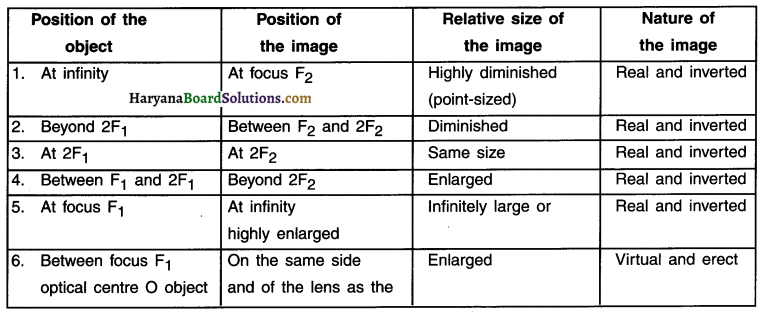Activity 13.

Aim: To locate the position and examine the nature of the image formed by a concave lens for different positions of the object.

1. Take a concave lens. Place it on a lens stand.
2. Place a burning candle on one side of the lens.
3. Look through the lens from the other side and observe the image. Try to get the image on a screen, if possible. If not, observe the image directly through the lens.
4. Note down the nature, relative size and approximate position of the image.
5. Move the candle away from the lens. Note the change In the size of the image. What happens to the size of the image when the candle is placed too far away from the lens.Observation and Conclusion:

• On observing the image directly through the lens, we can see that the image of the candle is virtual, erect, smaller than the candle and closer to the lens.
• On moving the candle away from the lens, the size of the image decreases.
• When the candle is placed very far from the lens, the image becomes highly diminished and appears point-like.